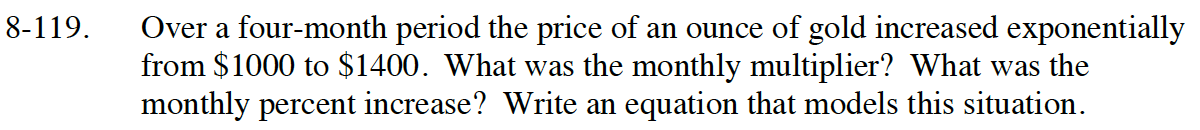### Home > INT1 > Chapter Ch8 > Lesson 8.2.3 > Problem8-119

8-119.

Over a four-month period the price of an ounce of gold increased exponentially from $1000 to$1400. What was the monthly multiplier? What was the monthly percent increase? Write an equation that models this situation. Homework Help ✎First, ask yourself these questions: What was the initial cost of an ounce of gold? The new price?
How long did it take for the gold to reach the new price?

If m is the monthly multiplier, then 1000(m)(m)(m)(m) = 1400.

The monthly multiplier is 1.088, and the monthly percent increase is 8.8%.